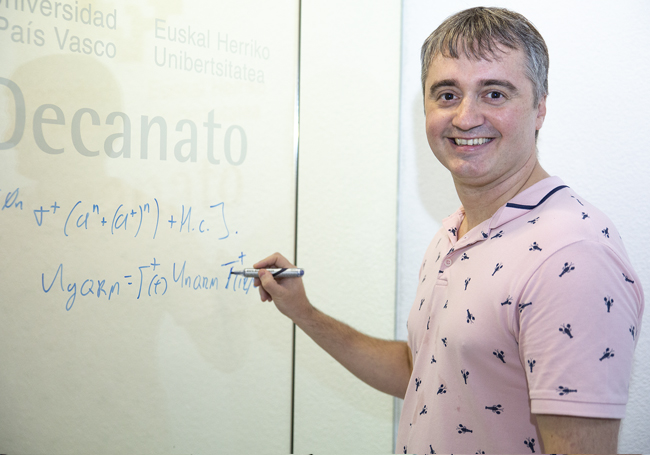Euskara español English

# A simple algorithm to speed up the processing of quantum information

A piece of work by a researcher at the UPV/EHU-University of the Basque Country shows that sophisticated mathematical models that are used to describe very different physical phenomena are integrated into the simple quantum Rabi model

• Ikerketa

First publication date: 04/10/2018

By means of a simple mathematical algorithm a researcher in the UPV/EHU’s Department of Physical Chemistry has studied the connection between various mathematical models, which were thought to be very different, for the purpose of speeding up the processing of quantum information. The prestigious international journal Npj Quantum Information has just published the results of this research.Jorge Casanova, researcher in the UPV/EHU’s Department of Physical Chemistry. Photo: UPV/EHU

Quantum mechanics has revolutionised the world of communications and computers with the introduction of algorithms that are much faster and much more secure when information is transferred. “The quantum Rabi model is a fundamental model that appears in many physical systems, including quantum platforms such as trapped ions or superconductor circuits, which in the fairly near future could be used as hardware in a quantum computer,” explained Jorge Casanova, a member of the Quantum Technologies for Information Science (QUTIS) research group at the UPV/EHU’s Department of Physical Chemistry and the author of the work. “It is the simplest mathematical model we have to describe the interaction processes between light and matter. In other words, as the UPV/EHU researcher pointed out, “the quantum Rabi model is the mechanism that matter and light (radiation) have selected in order to communicate with each other.”

This work has shown for the first time that various, highly sophisticated mathematical models which are used to describe various physical phenomena are found within the same quantum Rabi model. “It is as if the quantum Rabi model were the root of a much more advanced set of mathematical models,” said Casanova. “This means that if you interpret the results predicted by the quantum Rabi model, you would have direct access to the predictions of other more complex models,” pointed out the researcher. Basically, “we have connected the quantum Rabi model with other more sophisticated mathematical models that were thought to be very different, all by means of one mathematical algorithm, in other words, through a series of rules that are also fairly simple,” he added.

So “a deeper understanding of the operational mechanism of the quantum Rabi model would enable us, firstly, to more precisely tackle the technical problems involved in the development of a quantum computer”, stressed the author of the work.  Secondly, “given that we would now gain direct access to other models beyond the quantum Rabi model,” said Casanova, “we would also gain direct access for the purposes of changing the most basic operational mechanism of the above-mentioned quantum platforms, and therefore of a future quantum computer”.

Jorge Casanova went on to point out that “beyond the possible practical applications that may emerge and which are undoubtedly of huge interest, it strikes me as a physicist incredible that such a simple model should continue to withhold secrets like the one we have discovered. It is an unexpected step forward, from which we still have to learn and extract all the possible information so that we can open up new channels of development in the field of quantum computing,” added the UPV/EHU researcher.

About the QUTIS group

Jorge Casanova worked at the University of Ulm (Germany) and right now is a Juan de la Cierva researcher in the QUTIS Group at the UPV/EHU. The QUTIS Group is a world leader in quantum simulation and computation and has come up with proposals for quantum implementations that have been carried by the best labs across the world. It collaborates with researchers across the world and works in a range of quantum platforms, such as trapped ions, superconductor circuits, quantum photonics, and nuclear magnetic resonance.